Скачать презентацию Work in Fluid Systems Both liquids and gases

a7b75f902012a4d3bc5d9663b7589b2b.ppt

• Количество слайдов: 14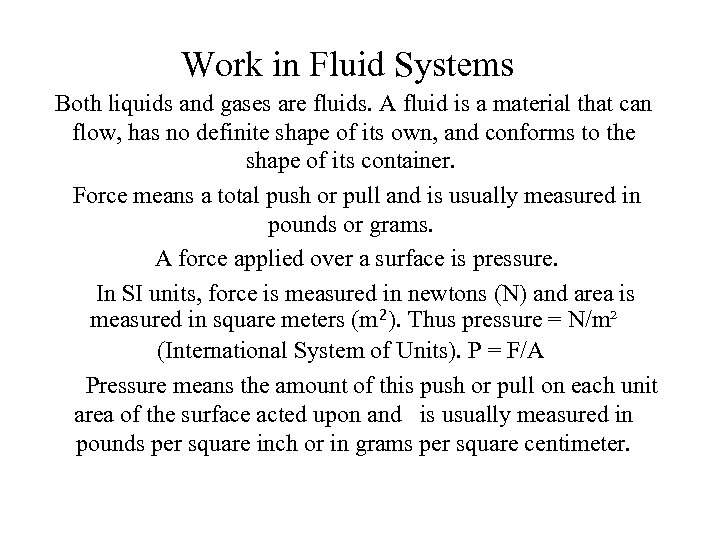Work in Fluid Systems Both liquids and gases are fluids. A fluid is a material that can flow, has no definite shape of its own, and conforms to the shape of its container. Force means a total push or pull and is usually measured in pounds or grams. A force applied over a surface is pressure. In SI units, force is measured in newtons (N) and area is measured in square meters (m²). Thus pressure = N/m² (International System of Units). P = F/A Pressure means the amount of this push or pull on each unit area of the surface acted upon and is usually measured in pounds per square inch or in grams per square centimeter.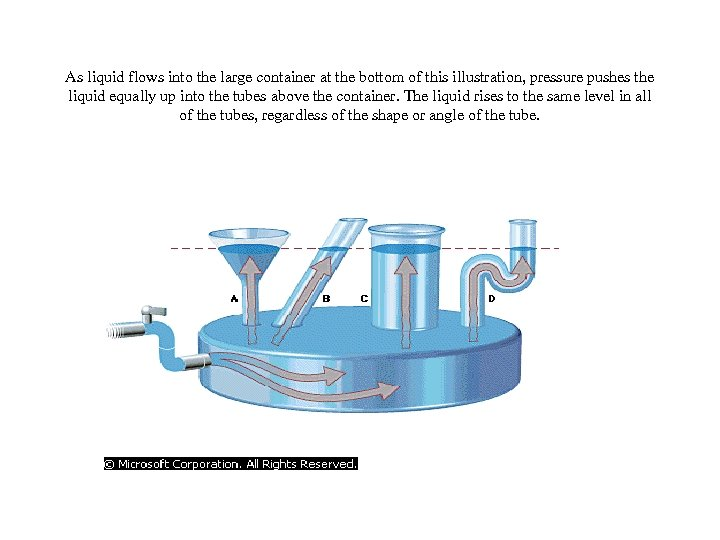As liquid flows into the large container at the bottom of this illustration, pressure pushes the liquid equally up into the tubes above the container. The liquid rises to the same level in all of the tubes, regardless of the shape or angle of the tube.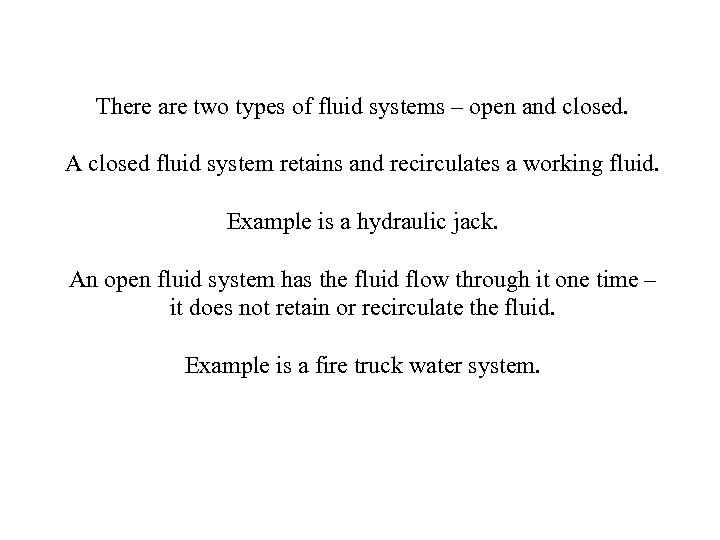There are two types of fluid systems – open and closed. A closed fluid system retains and recirculates a working fluid. Example is a hydraulic jack. An open fluid system has the fluid flow through it one time – it does not retain or recirculate the fluid. Example is a fire truck water system.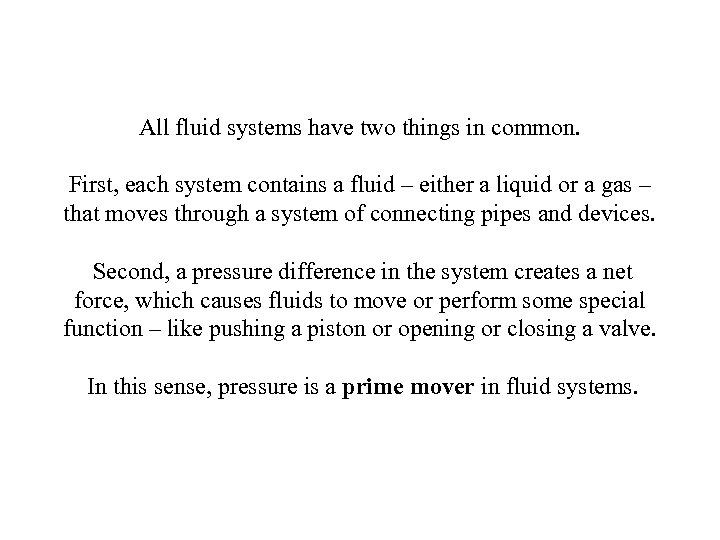All fluid systems have two things in common. First, each system contains a fluid – either a liquid or a gas – that moves through a system of connecting pipes and devices. Second, a pressure difference in the system creates a net force, which causes fluids to move or perform some special function – like pushing a piston or opening or closing a valve. In this sense, pressure is a prime mover in fluid systems.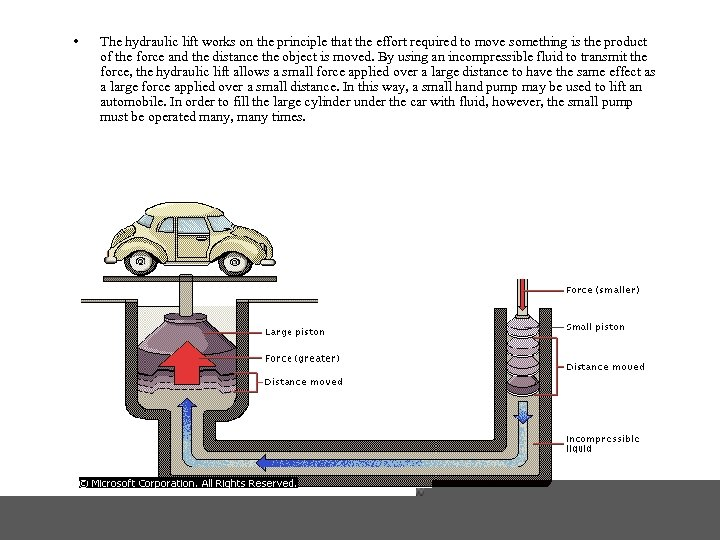• The hydraulic lift works on the principle that the effort required to move something is the product of the force and the distance the object is moved. By using an incompressible fluid to transmit the force, the hydraulic lift allows a small force applied over a large distance to have the same effect as a large force applied over a small distance. In this way, a small hand pump may be used to lift an automobile. In order to fill the large cylinder under the car with fluid, however, the small pump must be operated many, many times.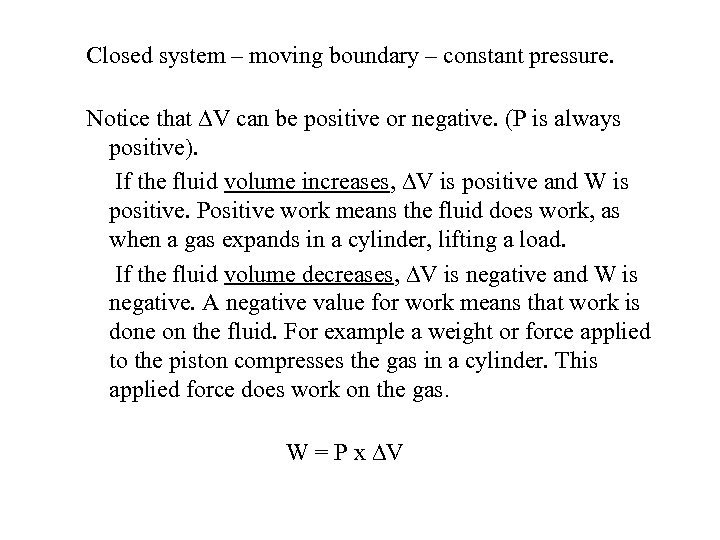Closed system – moving boundary – constant pressure. Notice that V can be positive or negative. (P is always positive). If the fluid volume increases, V is positive and W is positive. Positive work means the fluid does work, as when a gas expands in a cylinder, lifting a load. If the fluid volume decreases, V is negative and W is negative. A negative value for work means that work is done on the fluid. For example a weight or force applied to the piston compresses the gas in a cylinder. This applied force does work on the gas. W = P x V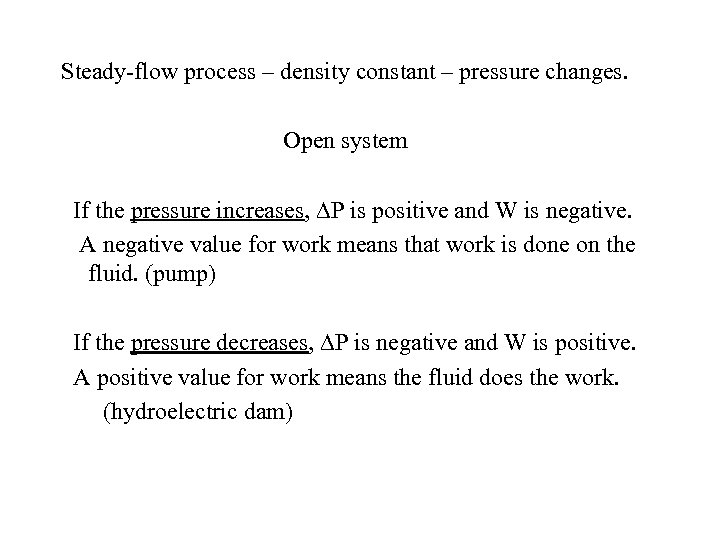Steady-flow process – density constant – pressure changes. Open system If the pressure increases, P is positive and W is negative. A negative value for work means that work is done on the fluid. (pump) If the pressure decreases, P is negative and W is positive. A positive value for work means the fluid does the work. (hydroelectric dam)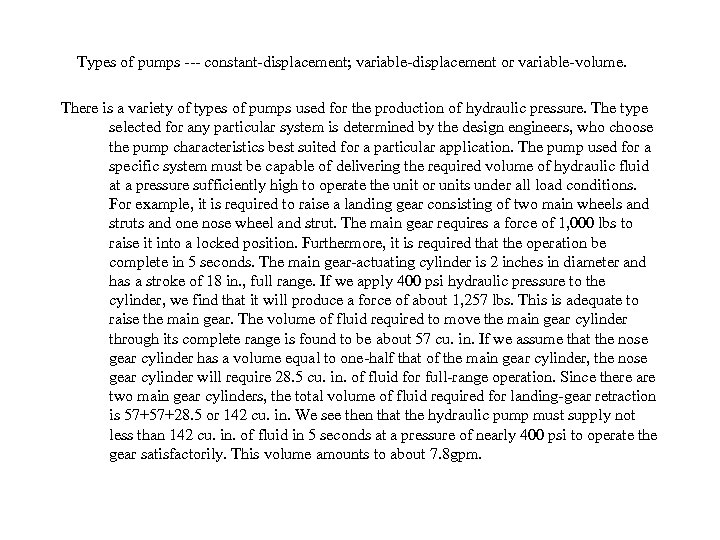Types of pumps --- constant-displacement; variable-displacement or variable-volume. There is a variety of types of pumps used for the production of hydraulic pressure. The type selected for any particular system is determined by the design engineers, who choose the pump characteristics best suited for a particular application. The pump used for a specific system must be capable of delivering the required volume of hydraulic fluid at a pressure sufficiently high to operate the unit or units under all load conditions. For example, it is required to raise a landing gear consisting of two main wheels and struts and one nose wheel and strut. The main gear requires a force of 1, 000 lbs to raise it into a locked position. Furthermore, it is required that the operation be complete in 5 seconds. The main gear-actuating cylinder is 2 inches in diameter and has a stroke of 18 in. , full range. If we apply 400 psi hydraulic pressure to the cylinder, we find that it will produce a force of about 1, 257 lbs. This is adequate to raise the main gear. The volume of fluid required to move the main gear cylinder through its complete range is found to be about 57 cu. in. If we assume that the nose gear cylinder has a volume equal to one-half that of the main gear cylinder, the nose gear cylinder will require 28. 5 cu. in. of fluid for full-range operation. Since there are two main gear cylinders, the total volume of fluid required for landing-gear retraction is 57+57+28. 5 or 142 cu. in. We see then that the hydraulic pump must supply not less than 142 cu. in. of fluid in 5 seconds at a pressure of nearly 400 psi to operate the gear satisfactorily. This volume amounts to about 7. 8 gpm.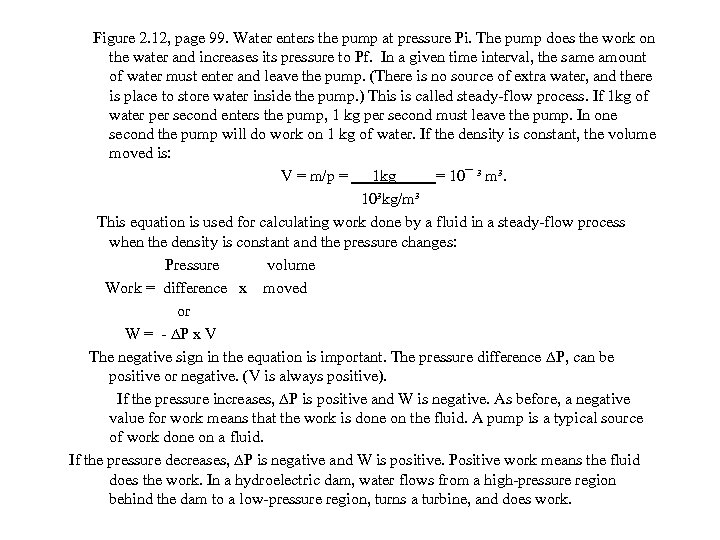Figure 2. 12, page 99. Water enters the pump at pressure Pi. The pump does the work on the water and increases its pressure to Pf. In a given time interval, the same amount of water must enter and leave the pump. (There is no source of extra water, and there is place to store water inside the pump. ) This is called steady-flow process. If 1 kg of water per second enters the pump, 1 kg per second must leave the pump. In one second the pump will do work on 1 kg of water. If the density is constant, the volume moved is: V = m/p = 1 kg = 10¯ ³ m³. 10³kg/m³ This equation is used for calculating work done by a fluid in a steady-flow process when the density is constant and the pressure changes: Pressure volume Work = difference x moved or W = - P x V The negative sign in the equation is important. The pressure difference P, can be positive or negative. (V is always positive). If the pressure increases, P is positive and W is negative. As before, a negative value for work means that the work is done on the fluid. A pump is a typical source of work done on a fluid. If the pressure decreases, P is negative and W is positive. Positive work means the fluid does the work. In a hydroelectric dam, water flows from a high-pressure region behind the dam to a low-pressure region, turns a turbine, and does work.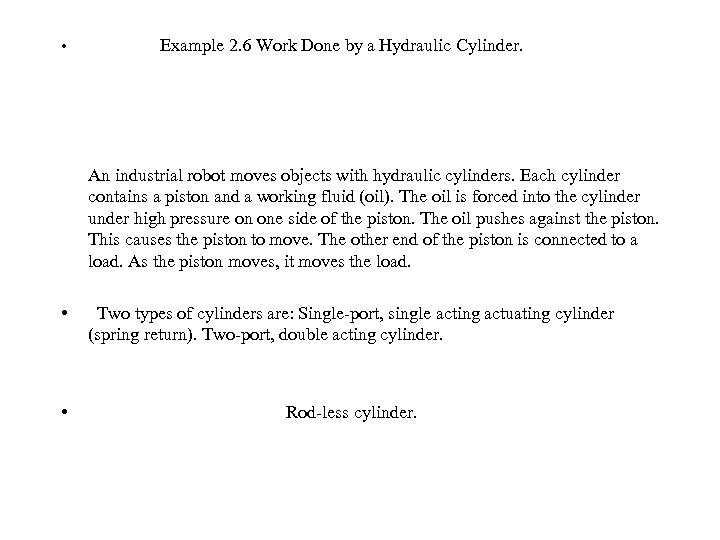• Example 2. 6 Work Done by a Hydraulic Cylinder. An industrial robot moves objects with hydraulic cylinders. Each cylinder contains a piston and a working fluid (oil). The oil is forced into the cylinder under high pressure on one side of the piston. The oil pushes against the piston. This causes the piston to move. The other end of the piston is connected to a load. As the piston moves, it moves the load. • Two types of cylinders are: Single-port, single acting actuating cylinder (spring return). Two-port, double acting cylinder. • Rod-less cylinder.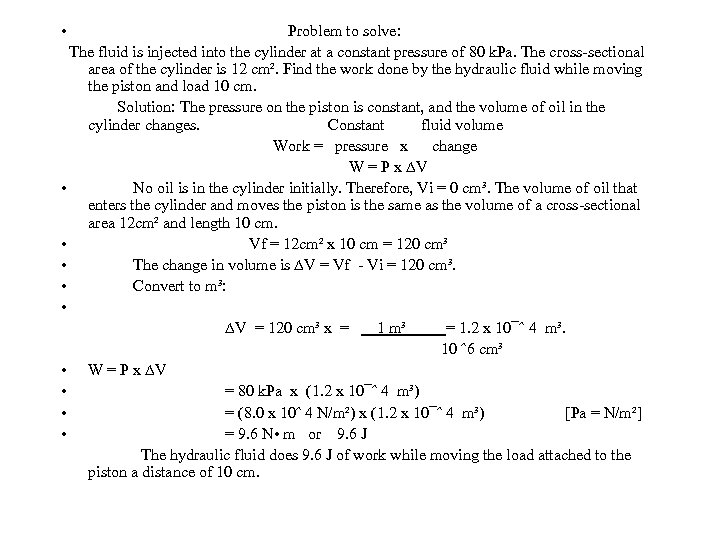• Problem to solve: The fluid is injected into the cylinder at a constant pressure of 80 k. Pa. The cross-sectional area of the cylinder is 12 cm². Find the work done by the hydraulic fluid while moving the piston and load 10 cm. Solution: The pressure on the piston is constant, and the volume of oil in the cylinder changes. Constant fluid volume Work = pressure x change W = P x V • No oil is in the cylinder initially. Therefore, Vi = 0 cm³. The volume of oil that enters the cylinder and moves the piston is the same as the volume of a cross-sectional area 12 cm² and length 10 cm. • Vf = 12 cm² x 10 cm = 120 cm³ • The change in volume is V = Vf - Vi = 120 cm³. • Convert to m³: • V = 120 cm³ x = 1 m³ = 1. 2 x 10¯ˆ 4 m³. 10 ˆ6 cm³ • W = P x V • = 80 k. Pa x (1. 2 x 10¯ˆ 4 m³) • = (8. 0 x 10ˆ 4 N/m²) x (1. 2 x 10¯ˆ 4 m³) [Pa = N/m²] • = 9. 6 N • m or 9. 6 J The hydraulic fluid does 9. 6 J of work while moving the load attached to the piston a distance of 10 cm.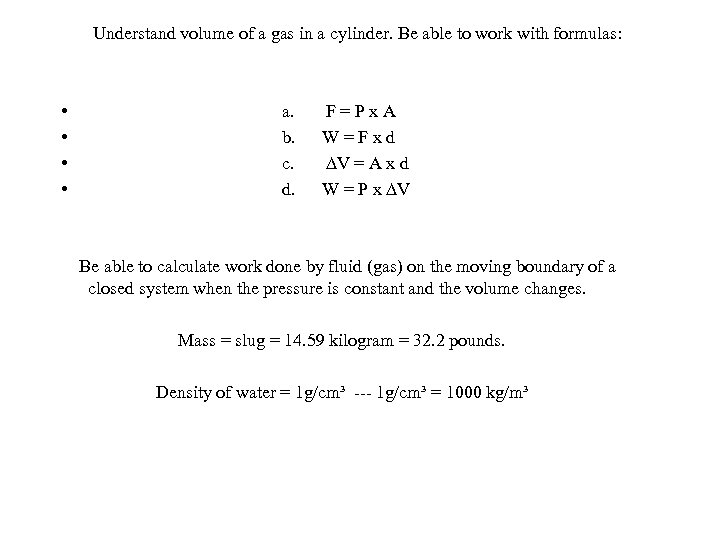Understand volume of a gas in a cylinder. Be able to work with formulas: • • a. F = P x A b. W = F x d c. V = A x d d. W = P x V Be able to calculate work done by fluid (gas) on the moving boundary of a closed system when the pressure is constant and the volume changes. Mass = slug = 14. 59 kilogram = 32. 2 pounds. Density of water = 1 g/cm³ --- 1 g/cm³ = 1000 kg/m³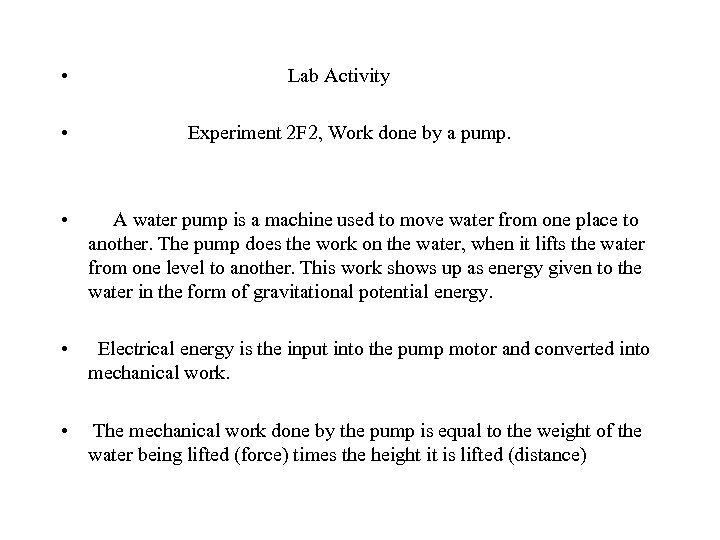• Lab Activity • Experiment 2 F 2, Work done by a pump. • A water pump is a machine used to move water from one place to another. The pump does the work on the water, when it lifts the water from one level to another. This work shows up as energy given to the water in the form of gravitational potential energy. • Electrical energy is the input into the pump motor and converted into mechanical work. • The mechanical work done by the pump is equal to the weight of the water being lifted (force) times the height it is lifted (distance)end